# travelingsalesmanproblem-170122053648.pdf

26 de Mar de 2023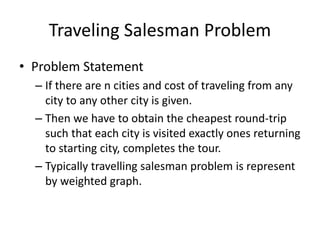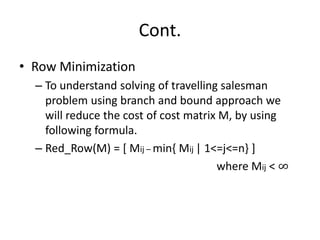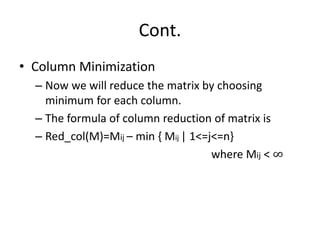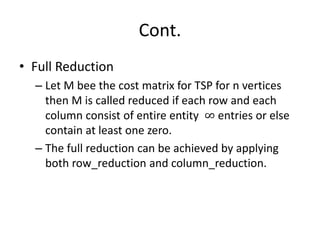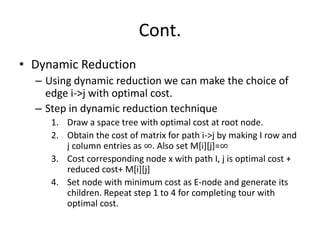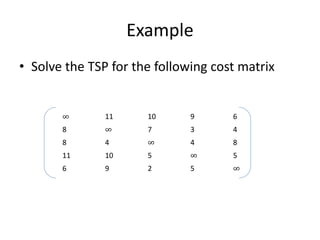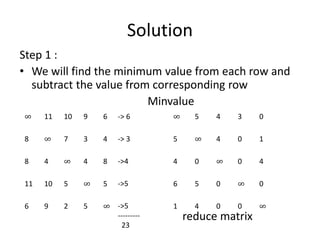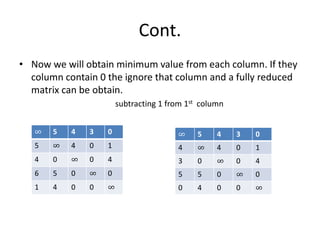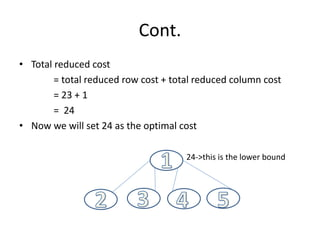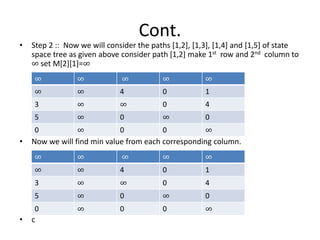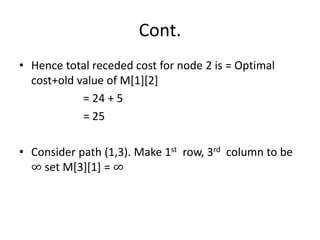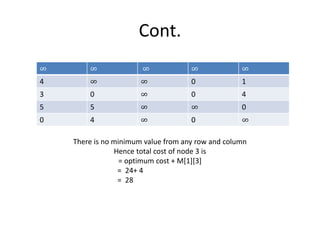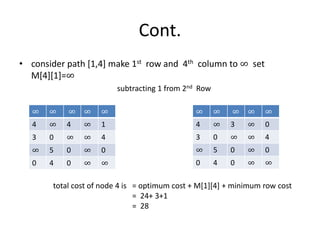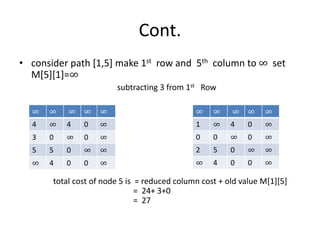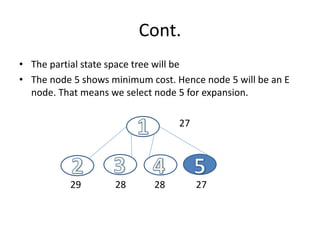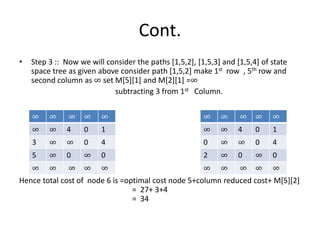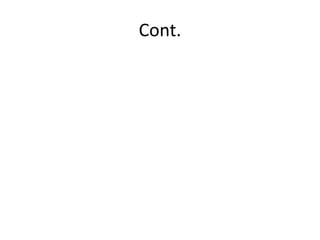1 de 17

### travelingsalesmanproblem-170122053648.pdf

• 1. Traveling Salesman Problem • Problem Statement – If there are n cities and cost of traveling from any city to any other city is given. – Then we have to obtain the cheapest round-trip such that each city is visited exactly ones returning to starting city, completes the tour. – Typically travelling salesman problem is represent by weighted graph.
• 2. Cont. • Row Minimization – To understand solving of travelling salesman problem using branch and bound approach we will reduce the cost of cost matrix M, by using following formula. – Red_Row(M) = [ Mij – min{ Mij | 1<=j<=n} ] where Mij < ∞
• 3. Cont. • Column Minimization – Now we will reduce the matrix by choosing minimum for each column. – The formula of column reduction of matrix is – Red_col(M)=Mij – min { Mij | 1<=j<=n} where Mij < ∞
• 4. Cont. • Full Reduction – Let M bee the cost matrix for TSP for n vertices then M is called reduced if each row and each column consist of entire entity ∞ entries or else contain at least one zero. – The full reduction can be achieved by applying both row_reduction and column_reduction.
• 5. Cont. • Dynamic Reduction – Using dynamic reduction we can make the choice of edge i->j with optimal cost. – Step in dynamic reduction technique 1. Draw a space tree with optimal cost at root node. 2. Obtain the cost of matrix for path i->j by making I row and j column entries as ∞. Also set M[i][j]=∞ 3. Cost corresponding node x with path I, j is optimal cost + reduced cost+ M[i][j] 4. Set node with minimum cost as E-node and generate its children. Repeat step 1 to 4 for completing tour with optimal cost.
• 6. Example • Solve the TSP for the following cost matrix ∞ 11 10 9 6 8 ∞ 7 3 4 8 4 ∞ 4 8 11 10 5 ∞ 5 6 9 2 5 ∞
• 7. Solution Step 1 : • We will find the minimum value from each row and subtract the value from corresponding row Minvalue reduce matrix ∞ 11 10 9 6 8 ∞ 7 3 4 8 4 ∞ 4 8 11 10 5 ∞ 5 6 9 2 5 ∞ ∞ 5 4 3 0 5 ∞ 4 0 1 4 0 ∞ 0 4 6 5 0 ∞ 0 1 4 0 0 ∞ -> 6 -> 3 ->4 ->5 ->5 --------- 23
• 8. Cont. • Now we will obtain minimum value from each column. If they column contain 0 the ignore that column and a fully reduced matrix can be obtain. subtracting 1 from 1st column ∞ 5 4 3 0 5 ∞ 4 0 1 4 0 ∞ 0 4 6 5 0 ∞ 0 1 4 0 0 ∞ ∞ 5 4 3 0 4 ∞ 4 0 1 3 0 ∞ 0 4 5 5 0 ∞ 0 0 4 0 0 ∞
• 9. Cont. • Total reduced cost = total reduced row cost + total reduced column cost = 23 + 1 = 24 • Now we will set 24 as the optimal cost 24->this is the lower bound
• 10. Cont. • Step 2 :: Now we will consider the paths [1,2], [1,3], [1,4] and [1,5] of state space tree as given above consider path [1,2] make 1st row and 2nd column to ∞ set M=∞ • Now we will find min value from each corresponding column. • c ∞ ∞ ∞ ∞ ∞ ∞ ∞ 4 0 1 3 ∞ ∞ 0 4 5 ∞ 0 ∞ 0 0 ∞ 0 0 ∞ ∞ ∞ ∞ ∞ ∞ ∞ ∞ 4 0 1 3 ∞ ∞ 0 4 5 ∞ 0 ∞ 0 0 ∞ 0 0 ∞
• 11. Cont. • Hence total receded cost for node 2 is = Optimal cost+old value of M = 24 + 5 = 25 • Consider path (1,3). Make 1st row, 3rd column to be ∞ set M = ∞
• 12. Cont. There is no minimum value from any row and column Hence total cost of node 3 is = optimum cost + M = 24+ 4 = 28 ∞ ∞ ∞ ∞ ∞ 4 ∞ ∞ 0 1 3 0 ∞ 0 4 5 5 ∞ ∞ 0 0 4 ∞ 0 ∞
• 13. Cont. • consider path [1,4] make 1st row and 4th column to ∞ set M=∞ subtracting 1 from 2nd Row total cost of node 4 is = optimum cost + M + minimum row cost = 24+ 3+1 = 28 ∞ ∞ ∞ ∞ ∞ 4 ∞ 4 ∞ 1 3 0 ∞ ∞ 4 ∞ 5 0 ∞ 0 0 4 0 ∞ ∞ ∞ ∞ ∞ ∞ ∞ 4 ∞ 3 ∞ 0 3 0 ∞ ∞ 4 ∞ 5 0 ∞ 0 0 4 0 ∞ ∞
• 14. Cont. • consider path [1,5] make 1st row and 5th column to ∞ set M=∞ subtracting 3 from 1st Row total cost of node 5 is = reduced column cost + old value M = 24+ 3+0 = 27 ∞ ∞ ∞ ∞ ∞ 4 ∞ 4 0 ∞ 3 0 ∞ 0 ∞ 5 5 0 ∞ ∞ ∞ 4 0 0 ∞ ∞ ∞ ∞ ∞ ∞ 1 ∞ 4 0 ∞ 0 0 ∞ 0 ∞ 2 5 0 ∞ ∞ ∞ 4 0 0 ∞
• 15. Cont. • The partial state space tree will be • The node 5 shows minimum cost. Hence node 5 will be an E node. That means we select node 5 for expansion. 27 29 28 28 27
• 16. Cont. • Step 3 :: Now we will consider the paths [1,5,2], [1,5,3] and [1,5,4] of state space tree as given above consider path [1,5,2] make 1st row , 5th row and second column as ∞ set M and M =∞ subtracting 3 from 1st Column. Hence total cost of node 6 is =optimal cost node 5+column reduced cost+ M = 27+ 3+4 = 34 ∞ ∞ ∞ ∞ ∞ ∞ ∞ 4 0 1 3 ∞ ∞ 0 4 5 ∞ 0 ∞ 0 ∞ ∞ ∞ ∞ ∞ ∞ ∞ ∞ ∞ ∞ ∞ ∞ 4 0 1 0 ∞ ∞ 0 4 2 ∞ 0 ∞ 0 ∞ ∞ ∞ ∞ ∞
• 17. Cont.